# NCERT Solutions for Class 10 Maths Chapter 15 Probability Ex 15.1

NCERT Solutions for Class 10 Maths Chapter 15 Probability Ex 15.1 are part of NCERT Solutions for Class 10 Maths. Here we have given NCERT Solutions for Class 10 Maths Chapter 15 Probability Ex 15.1. https://mcqquestions.guru/ncert-solutions-for-class-10-maths-chapter-15/

 Board CBSE Textbook NCERT Class Class 10 Subject Maths Chapter Chapter 15 Chapter Name Probability Exercise Ex 15.1 Number of Questions Solved 25 Category NCERT Solutions

## NCERT Solutions for Class 10 Maths Chapter 15 Probability Ex 15.1

Question 1.
Complete the following statements:
(i) Probability of an event E + Probability of the event ‘not E’ = ………
(ii) The probability of an event that cannot happen is ……… Such an event is called ………
(iii) The probability of an event that is certain to happen is ………. Such an event is called ………
(iv) The sum of the probabilities of all the elementary events of an experiment is ………..
(v) The probability of an event is greater than or equal to …………. and less than or equal to ………..
Solution:
(i) Probability of an event E + Probability of the event ‘not E’ = 1.
(ii) The probability of an event that cannot happen is 0. Such an event is called impossible event.
(iii) The probability of an event that is certain to happen is 1. Such an event is called sure event.
(iv) The sum of the probabilities of all the elementary events of an experiment is 1.
(v) The probability of an event is greater than or equal to 0 and less than or equal to 1.

Question 2.
Which of the following experiments have equally likely outcomes? Explain.
(i) A driver attempts to start a car. The car starts or does not start.
(ii) A player attempts to shoot a basketball. She/he shoots or misses the shot.
(iv) A baby is born. It is a boy or a girl.
Solution:
(i) The outcome is not equally likely because the car starts normally only when there is some defect, the car does not start.
(ii) The outcome is not equally likely because the outcome depends on the training of the player.
(iii) The outcome in the trial of true-false question is, either true or false. Hence, the two outcomes are equally likely.
(iv) A baby can be either a boy or a girl and both the outcomes have equally likely chances.

Question 3.
Why is tossing a coin considered to be a fair way of deciding which team should get the bail at the beginning of a football game?
Solution:
When we toss a coin, the outcomes head and tail are equally likely. So, the result of an individual coin toss is completely unpredictable.

Question 4.
Which of the following cannot be the probability of an event?
(A) $$\frac { 2 }{ 3 }$$
(B) -1.5
(C) 15%
(D) 0.7
Solution:
We know that probability of an event cannot be less than 0 and greater than 1.
Correct option is (B).

Question 5.
If P (E) = 0.05, what is the probability of ‘not E’?
Solution:
We have, P (E) + P (not E) = 1
Given: P(E) = 0.05
P (not E) = 1 – 0.05 = 0.95

Question 6.
A bag contains lemon flavoured candies only. Malini takes out one candy without looking into the bag. What is the probability that she takes out
(i) an orange flavoured candy?
(ii) a lemon flavoured candy?
Solution:
(i) A bag contains only lemon flavoured candies.
P (an orange flavoured candy) = 0
(ii) P (a lemon flavoured candy) = 1

Question 7.
It is given that in a group of 3 students, the probability of 2 students not having the same birthday is 0.992. What is the probability that the 2 students have the same birthday?
Solution:
We have, P (E) + P (not E) = 1
⇒ P (E) + 0.992 = 1
⇒ P (E) = 1 – 0.992 = 0.008

Question 8.
A bag contains 3 red balls and 5 black balls. A ball is drawn at random from the bag. What is the probability that the ball drawn is
(i) red?
(ii) not red?
Solution:
Number of red balls = 3
Number of black balls = 5
Total number of balls = 3 + 5 = 8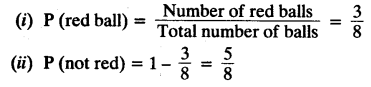Question 9.
A box contains 5 red marbles, 8 white marbles and 4 green marbles. One marble is taken out of the box at random. What is the probability that the marble taken out will be
(i) red?
(ii) white?
(iii) not green?
Solution:
Total number of marbles = 5 + 8 + 4 = 17
(i) P (red marble) = $$\frac { 5 }{ 17 }$$
(ii) P (white marble) = $$\frac { 8 }{ 17 }$$
(iii) P (not a green marble) = $$\frac { 13 }{ 17 }$$

Question 10.
A piggy bank contains hundred 50 p coins, fifty ₹ 1 coins, twenty ₹ 2 coins and ten ₹ 5 coins. If it is equally likely that one of the coins will fall out when the bank is turned upside down, what is the probability that the coin
(i) will be a 50 p coin?
(ii) will not be a ₹ 5 coin?
Solution:
Number of 50 p coins = 100
Number of ₹ 1 coins = 50
Number of ₹ 2 coins = 20
Number of ₹ 5 coins = 10
Total number of coins = 180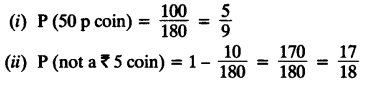Question 11.
Gopi buys a fish from a shop for his aquarium. The shopkeeper takes out one fish at random from a tank containing 5 male fish and 8 female fish (see figure). What is the probability that the fish taken out is a male fish?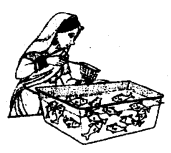Solution:
Number of male fish = 5
Number of female fish = 8
Total number of fish = 5 + 8 = 13
P (a male fish) = $$\frac { 5 }{ 13 }$$

Question 12.
A game of chance consists of spinning an arrow which comes to rest pointing at one of the numbers 1, 2, 3, 4, 5, 6, 7, 8 (see figure.), and these are equally likely outcomes. What is the probability that it will point at
(i) 8?
(ii) an odd number?
(iii) a number greater than 2?
(iv) a number less than 9?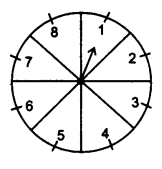Solution:
(i) P (getting 8) = $$\frac { 1 }{ 8 }$$
(ii) P (an odd number) = $$\frac { 4 }{ 8 }$$ = $$\frac { 1 }{ 2 }$$ ( odd numbers are 1, 3, 5, 7)
(iii) P (a number greater than 2) = $$\frac { 6 }{ 8 }$$ = $$\frac { 3 }{ 4 }$$
(iv) P (a number less than 9) = $$\frac { 8 }{ 8 }$$ = 1

Question 13.
A die is thrown once. Find the probability of getting
(i) a prime number
(ii) a number lying between 2 and 6
(ill) an odd number
Solution:
(i) Prime numbers on a die = 2, 3, 5
P (a prime number) = $$\frac { 3 }{ 6 }$$ = $$\frac { 1 }{ 2 }$$
(ii) Number lying between 2 and 6 = 3, 4, 5
P(a number lying between 2 and 6) = $$\frac { 3 }{ 6 }$$ = $$\frac { 1 }{ 2 }$$
(iii) Odd numbers = 1, 3, 5
P (an odd number) = $$\frac { 3 }{ 6 }$$ = $$\frac { 1 }{ 2 }$$

Question 14.
One card is drawn from a well-shuffled deck of 52 cards. Find the probability of getting
(i) a king of red colour
(ii) a face card
(iii) a red face card
(iv) the jack of hearts
(vi) the queen of diamonds
Solution:
Number of cards in a well-shuffled deck = 52.
(i) P (a king of red colour) = $$\frac { 2 }{ 52 }$$ = $$\frac { 1 }{ 26 }$$
(ii) P (a face card) = $$\frac { 12 }{ 52 }$$ = $$\frac { 3 }{ 13 }$$
(iii) P (a red face card) = $$\frac { 6 }{ 52 }$$ = $$\frac { 3 }{ 26 }$$
(iv) P (the jack of hearts) = $$\frac { 1 }{ 52 }$$
(v) P(a spade) = $$\frac { 13 }{ 52 }$$ = $$\frac { 1 }{ 4 }$$
(vi) P (the queen of diamonds) = $$\frac { 1 }{ 52 }$$

Question 15.
Five cards – the ten, jack, queen, king and ace of diamonds, are well shuffled with their face downwards. One card is then picked up at random.
(i) What is the probability that the card is the queen?
(ii) If the queen is drawn and put aside, what is the probability that the second card picked up is
(a) an ace?
(b) a queen?
Solution:
Out of 5 cards there is only one queen.
(i) P (getting queen) = $$\frac { 1 }{ 5 }$$ [when queen is drawn, four cards are left]
(ii) (a) P (an ace) = $$\frac { 1 }{ 4 }$$
(b) P (a queen) = $$\frac { 0 }{ 4 }$$ = 0

Question 16.
12 defective pens are accidentally mixed with 132 good ones. It is not possible to just look at a pen and tell whether or not it is defective. One pen is taken out at random from this lot. Determine the probability that the pen taken out is a good one.
Solution:
Number of defective pens = 12
Number of good pens = 132
Total number of pens = 12 + 132 = 144
P (the pen is good one) = $$\frac { 132 }{ 144 }$$ = $$\frac { 11 }{ 12 }$$

Question 17.
(i) A lot of 20 bulbs contain 4 defective ones. One bulb is drawn at random from the lot. What is the probability that this bulb is defective?
(ii) Suppose the bulb drawn in (i) is not defective and is not replaced. Now one bulb is drawn at random from the rest. What is the probability that this bulb is not defective?
Solution:
(i) Total number of bulbs = 20
Number of defective bulbs = 4
P (bulb drawn is defective) = $$\frac { 4 }{ 20 }$$ = $$\frac { 1 }{ 5 }$$
(ii) Remaining bulbs = 19
P (bulb drawn is not defective) = $$\frac { 15 }{ 19 }$$

Question 18.
A box contains 90 discs which are numbered from 1 to 90. If one disc is drawn at random from the box, find the probability that it bears
(i) a two digit number.
(ii) a perfect square number.
(iii) a number divisible by 5.
Solution:
Total numbers of discs = 90
(i) P (a two digit number) = $$\frac { 81 }{ 90 }$$ = $$\frac { 9 }{ 10 }$$
(ii) Here, perfect square numbers are 1, 4, 9, 16, 25, 36, 49, 64, 81
P (getting a perfect square number) = $$\frac { 9 }{ 90 }$$ = $$\frac { 1 }{ 10 }$$
(iii) Numbers divisible by 5 are 5, 10, 15, 20, 25, 30, 35, 40, 45, 50, 55, 60, 65, 70, 75, 80, 85, 90
P (getting a number divisible by 5) = $$\frac { 18 }{ 90 }$$ = $$\frac { 1 }{ 5 }$$

Question 19.
A child has a die whose six faces show the letters as given below: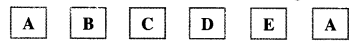The die is thrown once. What is the probability of getting
(i) A?
(ii) D?
Solution:
(i) P (getting A) = $$\frac { 2 }{ 6 }$$ = $$\frac { 1 }{ 3 }$$
(ii) P (getting D) = $$\frac { 1 }{ 6 }$$

Question 20.
Suppose you drop a die at random on the rectangular region shown in figure. What is the probability that it will land inside the circle with diameter 1 m?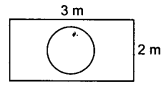Solution: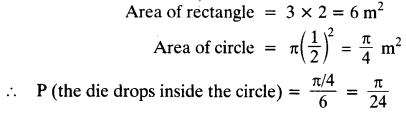Question 21.
A lot consists of 144 ball pens of which 20 are defective and the others are good. Nuri will buy a pen if it is good, but will not buy if it is defective. The shopkeeper draws one pen at random and gives it to her. What is the probability that
(ii) she will not buy it?
Solution:
Total number of ball pens = 144
Number of defective pens = 20
Number of good pens = 144 – 20 = 124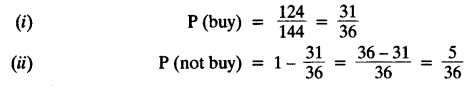Question 22.
Two dice, one blue and one grey, are thrown at the same time. Now
(i) Complete the following table: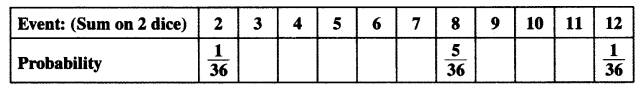(ii) A student argues that-there are 11 possible outcomes 2, 3, 4, 5, 6, 7, 8, 9, 10, 11 and 12. Therefore, each of them has a probability $$\frac { 1 }{ 11 }$$. Do you agree with this argument? Justify your answer.
Solution:
(i) Total number of possible outcomes = 36
(1, 2) and (2, 1) are the favourable events of getting the sum 3.
P(sum 3) = $$\frac { 2 }{ 36 }$$ = $$\frac { 1 }{ 18 }$$
(1, 3) , (2, 2) and (3, 1) are the favourable events of getting the sum 4.
P(sum 4) = $$\frac { 3 }{ 36 }$$ = $$\frac { 1 }{ 12 }$$
(1, 4) , (2, 3), (3, 2) and (4, 1) are the favourable events of getting the sum 5.
P(sum 5) = $$\frac { 4 }{ 36 }$$ = $$\frac { 1 }{ 9 }$$
(1, 5) , (2, 4), (3, 3), (4, 2) and (5, 1) are the favourable events of getting the sum 6.
P (sum 6) = $$\frac { 5 }{ 36 }$$
(1, 6) , (2, 5), (3, 4), (4, 3), (5, 2) and (6, 1) are the favourable events of getting the sum 7.
P(sum 7) = $$\frac { 6 }{ 36 }$$ = $$\frac { 1 }{ 6 }$$
(3, 6) , (4, 5), (5, 4) and (6, 3) are the favourable events of getting the sum 9.
P(sum 9) = $$\frac { 4 }{ 36 }$$ = $$\frac { 1 }{ 9 }$$
(4, 6) , (5, 5) and (6, 4) are the favourable events of getting the sum 10.
P(sum 10 = $$\frac { 3 }{ 36 }$$ = $$\frac { 1 }{ 12 }$$
(5,6) and (6,5) are the favourable events of getting the sum 11.
P(sum 11) = $$\frac { 2 }{ 36 }$$ = $$\frac { 1 }{ 18 }$$
(ii) No, because the outcomes as 11 different sum are not equally likely.

Question 23.
A game consists of tossing a one rupee coin 3 times and noting its outcome each time. Hanif wins if all the tosses give the same result, i.e. three heads or three tails, and loses otherwise. Calculate the probability that Hanif will lose the game.
Solution:
Possible outcomes are
HHH, TTT, HHT, HTH, THH, TTH, THT, HTT = 8
P (win the game) = $$\frac { 2 }{ 8 }$$ = $$\frac { 1 }{ 4 }$$
P (lose the game) = 1 – $$\frac { 1 }{ 4 }$$ = $$\frac { 3 }{ 4 }$$

Question 24.
A die is thrown twice. What is the probability that
(i) 5 will not come up either time?
(ii) 5 will come up at least once?
[Hint: Throwing a die twice and throwing two dice simultaneously are treated as the same experiment.]
Solution:
Total outcomes = 36
Number of outcomes in favour of 5 is (1, 5) (2, 5) (3, 5) (4, 5) (5, 5) (6, 5) (5, 1) (5, 2) (5, 3) (5, 4) (5, 6) = 11
(i) P (5 will not come up either time) = $$\frac { 25 }{ 36 }$$
(ii) P (5 will come up at least once) = $$\frac { 11 }{ 36 }$$

Question 25.
Which of the following arguments are correct and which are not correct? Give reasons for your answer.
(i) If two coins are tossed simultaneously there are three possible outcomes- two heads, two tails or one of each. Therefore, for each of these outcomes, the probability is $$\frac { 1 }{ 3 }$$.
(ii) If a die is thrown, there are two possible outcomes- an odd number or an even number. Therefore, the probability of getting an odd number is $$\frac { 1 }{ 2 }$$.
Solution:
(i) Argument is incorrect.
The possible outcomes are (HH), (HT), (TH), (TT)
P(HH) = $$\frac { 1 }{ 4 }$$
P(TT) = $$\frac { 1 }{ 4 }$$
P(HT or TH) = $$\frac { 2 }{ 4 }$$ = $$\frac { 1 }{ 2 }$$
(ii) Argument is correct.
Possible outcomes = 1, 2, 3, 4, 5, 6
Odd numbers are = 1, 3, 5
P (an odd number) = $$\frac { 3 }{ 6 }$$ = $$\frac { 1 }{ 2 }$$

We hope the NCERT Solutions for Class 10 Maths Chapter 15 Probability Ex 15.1, help you. If you have any query regarding NCERT Solutions for Class 10 Maths Chapter 15 Probability Ex 15.1, drop a comment below and we will get back to you at the earliest.